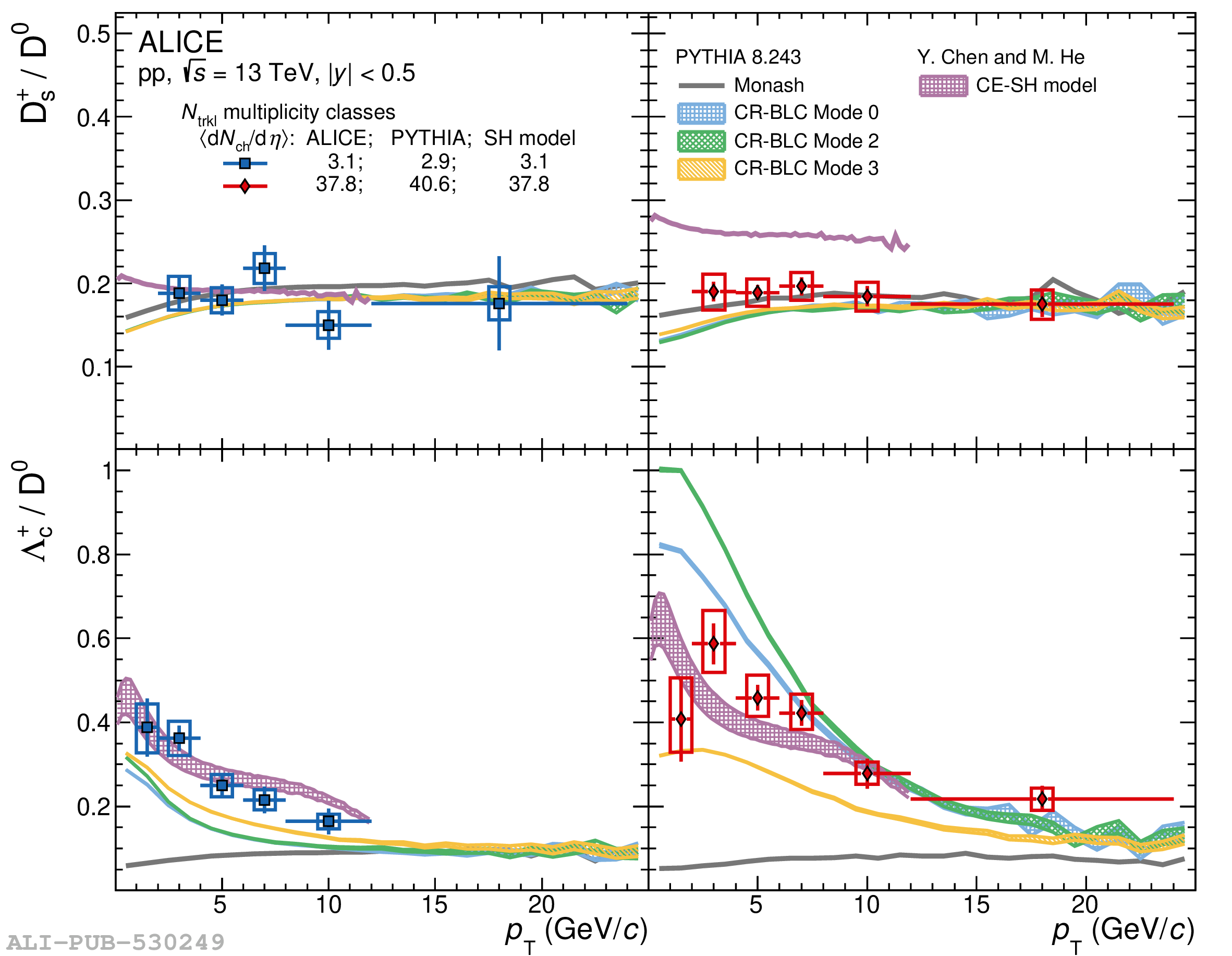# Figure 3

 The ${\rm D_s^+}/{\rm D^0}$ (top) and $\Lambda_{\rm c}^+/{\rm D^0}$ (bottom) ratios measured in pp collisions at $\sqrt{s}=13$ TeV for the lowest (left) and highest (right) multiplicity classes at midrapidity. The measurements are compared to PYTHIA predictions with the Monash  and the CR-BLC tunes , and the CE-SH model , estimated in similar multiplicity classes. The uncertainty bands for the PYTHIA predictions are the statistical uncertainties on the simulations, while for the CE-SH model they refer to the variation of the branching ratios of the additional charm-baryon states from RQM .